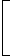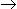# numbers aptitude questions

51. 476 ** 0 is divisible by both 3 and 11. The non-zero digits in the hundred’s and ten’s places are respectively:
 A. 7 and 4 B. 7 and 5 C. 8 and 5 D. None of these

Explanation:

Let the given number be 476 xy 0.

Then (4 + 7 + 6 + x + y + 0) = (17 + x + y) must be divisible by 3.

And, (0 + x + 7) – (y + 6 + 4) = (xy -3) must be either 0 or 11.

xy – 3 = 0y = x – 3

(17 + x + y) = (17 + x + x – 3) = (2x + 14)x= 2 or x = 8.x = 8 and y = 5.

52. If the number 97215 * 6 is completely divisible by 11, then the smallest whole number in place of * will be:
 A. 3 B. 2 C. 1 D. 5 E. None of these

53. (112 + 122 + 132 + … + 202) = ?
 A. 385 B. 2485 C. 2870 D. 3255

Explanation:

(112 + 122 + 132 + … + 202) = (12 + 22 + 32 + … + 202) – (12 + 22 + 32 + … + 102)Ref: (12 + 22 + 32 + … + n2) = 1 n(n + 1)(2n + 1)6
 =20 x 21 x 41 – 10 x 11 x 216 6

= (2870 – 385)

= 2485.

54. If the number 5 * 2 is divisible by 6, then * = ?
 A. 2 B. 3 C. 6 D. 7

Explanation:

6 = 3 x 2. Clearly, 5 * 2 is divisible by 2. Replace * by x.

Then, (5 + x + 2) must be divisible by 3. So, x = 2.

55. Which of the following numbers will completely divide (4915 – 1) ?
 A. 8 B. 14 C. 46 D. 50

Explanation:

(xn – 1) will be divisibly by (x + 1) only when n is even.

(4915 – 1) = {(72)15 – 1} = (730 – 1), which is divisible by (7 +1), i.e., 8.

56.

 9 + 3 + 7 + 2 –9 + 1= ? 4 17 15
A.
 7 + 719 1020
B.
 9 + 817 1020
C.
 9 + 719 1020
D.
 7 + 817 1020
E. None of these

Explanation:

Given sum
 = 9 + 3 + 7 + 2 –9 + 14 17 15
 = (9 + 7 – 9) +3 + 2 – 14 17 15
 = 7 + 765 + 120 – 68 1020
 = 7 + 817 1020

57.1 – 1+1 – 2+1 – 3+ … up to n terms = ? n n n
A.
 1 n 2
B.
 1 (n – 1) 2
C.
 1 n(n – 1) 2
D. None of these

58. On dividing 2272 as well as 875 by 3-digit number N, we get the same remainder. The sum of the digits of N is:
 A. 10 B. 11 C. 12 D. 13

Explanation:

Clearly, (2272 – 875) = 1397, is exactly divisible by N.

Now, 1397 = 11 x 127The required 3-digit number is 127, the sum of whose digits is 10.

59. A boy multiplied 987 by a certain number and obtained 559981 as his answer. If in the answer both 9 are wrong and the other digits are correct, then the correct answer would be:
 A. 553681 B. 555181 C. 555681 D. 556581

Explanation:

987 = 3 x 7 x 47

So, the required number must be divisible by each one of 3, 7, 47

553681(Sum of digits = 28, not divisible by 3)

555181(Sum of digits = 25, not divisible by 3)

555681 is divisible by 3, 7, 47.

60. How many prime numbers are less than 50 ?
 A. 16 B. 15 C. 14 D. 18